Courses

# Test: Thermodynamics 2 - From Past 28 Years Questions

## 30 Questions MCQ Test Physics 28 Years Past year papers for NEET/AIPMT Class 11 | Test: Thermodynamics 2 - From Past 28 Years Questions

Description
This mock test of Test: Thermodynamics 2 - From Past 28 Years Questions for NEET helps you for every NEET entrance exam. This contains 30 Multiple Choice Questions for NEET Test: Thermodynamics 2 - From Past 28 Years Questions (mcq) to study with solutions a complete question bank. The solved questions answers in this Test: Thermodynamics 2 - From Past 28 Years Questions quiz give you a good mix of easy questions and tough questions. NEET students definitely take this Test: Thermodynamics 2 - From Past 28 Years Questions exercise for a better result in the exam. You can find other Test: Thermodynamics 2 - From Past 28 Years Questions extra questions, long questions & short questions for NEET on EduRev as well by searching above.
QUESTION: 1

### First law of thermodynamics is consequence of conservation of 

Solution:

The first law of thermodynamics is just a conservation of energy.

QUESTION: 2

### At 27° Ca gas is compressed suddenly such that its pressure becomes (1/8) of original pressure. Final temperature will be (γ = 5/3)

Solution:

Temperature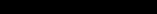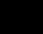Pressure becomes (1/8)th of its original pressure.

We know that,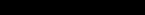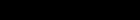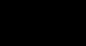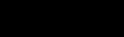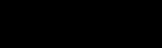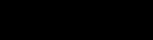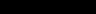QUESTION: 3

### A thermodynamic process is shown in the figure. The pressures and volumes corresponding to some points in the figure are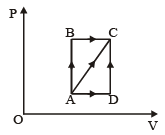PA = 3 × 104 Pa VA = 2 × 10-3 m3 PB = 8 × 104 Pa VD = 5 × 10–3 m3. In process AB, 600 J of heat is added to the system and in process BC, 200 J of heat is added to the system. The change in internal energy of the system in process AC would be 

Solution:

Since AB is an isochoric process, so, no work is done. BC is isobaric process,
∴ W = PB × (VD – VA) = 240 J
ΔQ = 600 + 200 = 800 J
Using ΔQ = ΔU + ΔW
⇒ ΔU = ΔQ – ΔW = 800 – 240 = 560 J

QUESTION: 4

For hydrogen gas, Cp – Cv = a and for oxygen gas, Cp – Cv = b, so the relation between a and b is given by 

Solution: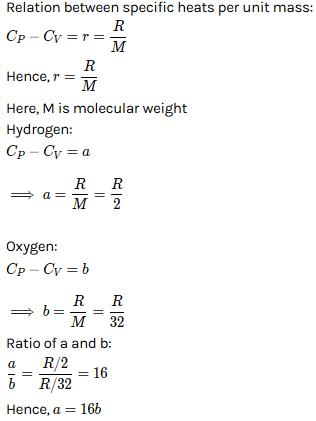QUESTION: 5

If for a gas,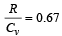, the gas is made up of molecules which are 

Solution:

Since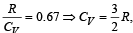hence gas is monoatomic.

QUESTION: 6

A thermodynamic system is taken from state A to B along ACB and is brought back to A along BDA  as shown in the PV diagram. The net work done during the complete cycle is given by the area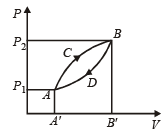Solution:

Work done = Area under curve ACBDA

QUESTION: 7

An ideal gas A and a real gas B have their volumes increased from V to 2V under isothermal conditions. The increase in internal energy 

Solution:

Under isothermal conditions, there is no change in internal energy.

QUESTION: 8

110 joules of heat is added to a gaseous system, whose internal energy is 40J; then the amount of external work done is 

Solution:

ΔQ = ΔU + ΔW
⇒   ΔW = ΔQ  – ΔU = 110 – 40 = 70 J

QUESTION: 9

Which of the following is not thermodynamical function ? 

Solution:

Work done is not a thermodynamical function.

QUESTION: 10

An ideal carnot engine, whose efficiency is 40% receives heat at 500 K. If its efficiency is 50%, then the intake temperature for the same exhaust temperature is 

Solution:

Efficiency of carnot engine (η1) = 40% = 0.4; Initial intake temperature (T1) = 500K and new efficiency (η2) = 50% = 0.5.
Efficiency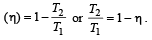Therefore in first case,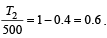⇒  T2 = 0.6×500=300K

And in second case,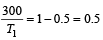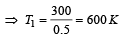QUESTION: 11

An ideal gas undergoing adiabatic change has the following pressure-temperature relationship 

Solution:

We know that in adiabatic process, PVγ = constant ....(1)
From ideal gas equation, we know that PV = nRT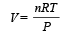....(2)
Puttingt the value from equation  (2) in equation (1),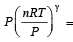constant

P(1 – γ) Tγ = constant

QUESTION: 12

A diatomic gas initially at 18ºC is compressed adiabatically to one eighth of its original volume.The temperature after compression will be 

Solution:

Initial temperature (T1) = 18°C = 291 K
Let Initial volume (V1) = V

Final volume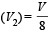According to adiabatic process, TVγ – 1 = constant According to question,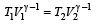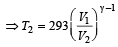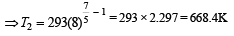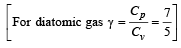QUESTION: 13

A sample of gas expands from volume V1 to V2.The amount of work done by the gas is greatest, when the expansion is 

Solution:

In thermodynamics for same change in volume, the work done is maximum in isobaric process because in P – V graph, area enclosed by curve and volume axis is maximum in isobaric process.
So, the choice (b) is correct.

QUESTION: 14

The efficiency of a Carn ot engine operating between the temperatures of 100ºC and –23ºC will be


Solution: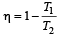T1 = –23°C = 250 K,   T2 = 100°C = 373K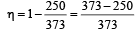QUESTION: 15

If the ratio of specific heat of a gas at constant pressure to that at constant volume is γ, the change in internal energy of a mass of gas, when the volume changes from V to 2V at constant pressure P, is 

Solution:

Change in internal energy is equal to work done in adiabatic system ΔW = –ΔU   (Expansion in the system)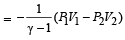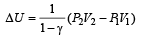Here,  V1 = V ,V2 = 2V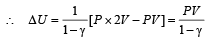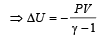QUESTION: 16

We consider a thermodynamic system. If ΔU represents the increase in its internal energy and W the work done by the system, which of the following statements is true? 

Solution:

ΔQ = ΔU + W
For adiabatic process, ΔQ = 0
ΔU = –W

QUESTION: 17

An ideal gas at 27ºC is compressed adiabatically to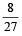of its original volume. The rise in temperature is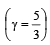Solution:

(T2/T1) = (V2/V1)γ-1
(T2/300) = (23/33)2/3
T2 = 675K
∆T = 375K

QUESTION: 18

A reversible engine converts one-sixth of the heat input into work. When the temperature of the sink is reduced by 62ºC, the efficiency of the engine is doubled. The temperatures of the source and sink are 

Solution:

Initially the efficiency of the engine was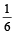which increases to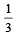when the sinktemperature reduces by 62º C.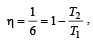when T2 = sink temperature T1 = source temperature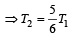Secondly,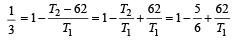or, T1 = 62 × 6 = 372K =372– 273 = 99ºC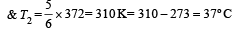QUESTION: 19

If γ be the ratio of specific heats of a perfect gas, the number of degrees of freedom of a molecule of the gas is 

Solution:

We know that ratio of specific heats,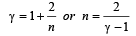[where n = Degree of freedom]

QUESTION: 20

The temperature of source and sink of a heat engine are 127ºC and 27ºC respectively. An inventor claims its efficiency to be 26%, then:

Solution: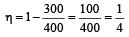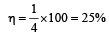Hence, it is not possible to have efficiency more than 25%.

QUESTION: 21

A gas at 27ºC temperature and 30 atmospheric pressure is allowed to expand to the atmospheric pressure. If the volume becomes 10 times its initial volume, then the final temperature becomes

Solution:

Given :  Initial temperature of gas (T1) = 27°C = 300 K
Initial pressure (P1) = 30 atm Initial volume (V1) = V
Final pressure (P2) = 1 atm Final volume (V2) = 10 V.
We know from the general gas  equation that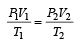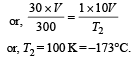QUESTION: 22

A Carnot engine whose efficiency is 50% has an exhaust temperature of 500 K. If the efficiency is to be 60% with the same intake temperature, the exhaust temperature must be (in K) 

Solution: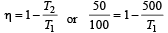⇒  T1 = 1000K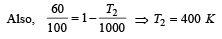QUESTION: 23

An ideal gas heat engine operates in a Carnot cycle between 227ºC and 127ºC. It absorbs 6 kcal at the higher temperature. The amount of heat (in kcal) converted into work is equal to

Solution:

Efficiency =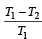T1 = 227 + 273 = 500 K
T2 = 127 + 273 = 400 K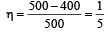Hence,  output work

= (η) Heat input =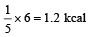QUESTION: 24

One mole of an ideal gas at an initial temperature of T K does 6R joules of work adiabatically. If the ratio of specific heats of this gas at constant pressure and at constant volume is 5/3, the final temperature of gas will be 

Solution:

T1 = T, W = 6R joules,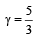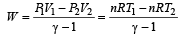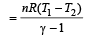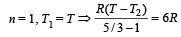⇒  T2 = ( T–4)K

QUESTION: 25

Which of the following processes is reversible?

Solution:

For a process to be reversible, it must be quasi-static. For quasi static process, all changes take place infinitely slowly.
Isothermal process occur very slowly so it is quasi-static and hence it is reversible.

QUESTION: 26

An ideal gas heat engine oper ates in Car not cycle between 227°C and 127°C. It absorbs 6 × 104 cals of heat at higher temperature. Amount of heat converted to work is 

Solution:

We know that efficiency of carnot engine =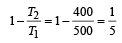[∵ T1 = (273 + 227)K = 500 K
and T2 = (273 + 127)K = 400 K]

Efficiency of Heat engine  =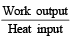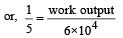⇒ work output = 1.2 × 104 cal

QUESTION: 27

A Carnot engine whose sink is at 300 K has an efficiency of 40%. By how much should the temperature of source be increased so as to increase, its efficiency by 50% of original efficiency ? 

Solution:

We know that efficiency of Carnot Engine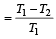where, T1 is temp. of source & T2 is temp. of sink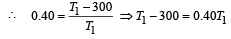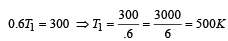Now efficiency to be increased by 50%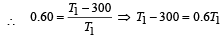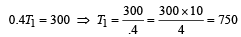Increase in temp = 750 – 500 = 250 K

QUESTION: 28

The molar specific heat at constant pressure of an ideal gas is (7/2) R. The ratio of specific heat at constant pressure to that at constant volume is

Solution: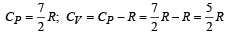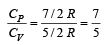QUESTION: 29

An engine has an efficiency of 1/6. When the temperature of sink is reduced by 62°C, its efficiency is doubled. Temperature of the source is

Solution:

Since efficiency of engine is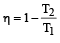According to problem,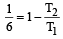.......... (1)

When the temperature of the sink is reduced by 62°C, its efficiency is doubled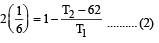Solving (1) and (2) T2 = 372K
T1 = 99°C = Temperature of source.

QUESTION: 30

If Q, E and W denote respectively the heat added, change in internal energy and the work done in a closed cyclic process, then: 

Solution:

In a cyclic process, the initial state coincides with the final state. Hence, the change in internal energy is zero, as it depends only on the initial and final states. But Q & W are non-zero during a cycle process.